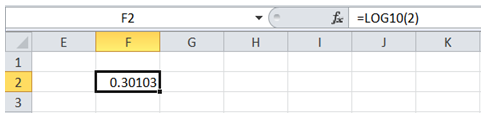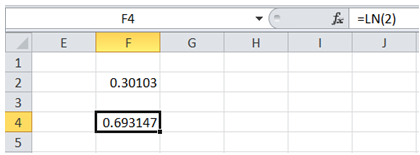# Excel Made Easy

## Get logarithms of value in Excel

To do it in Excel, here is the answer:

a) Enter the formula =LOG10(number), where number is the value for which log value is to be determined.b) In case natural logarithm of number is required (as opposed to base 10), enter the formula =LN(number), where number is the value for which log value is to be determined.